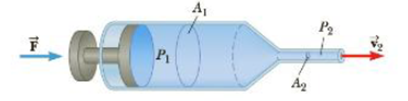Chapter 9, Problem 37P

Chapter
Section
Textbook Problem

A hypodermic syringe contain a medicine with the density of water (Fig. P9.37). The barrel of the syringe has a cross-sectional area of in the absence of a force on the plunger, the pressure everywhere is 1.00 atm. A force F → of magnitude 2.00 N is exerted on the plunger, making medicine squirt from the needle. Determine the medicine’s How speed through the needle. Assume the pressure in the needle remains equal to 1.00 atm and that the syringe is horizontal.Figure P9.37

To determine
The medicine’s flow speed through the needle.

Explanation
Assume that the level of syringe is at y=0 and use Bernoulli’s equation for the flow speed of the medicine such that P2+(1/2)ρv22+0=P1+(1/2)ρv12 , the rearrangement of this expression for the speed of medicine flow as v2=v12+(2(P1P2)/ρ) but the pressure difference at both ends would be P1P2=P1Patm=(P1)gauge=F/A1 . Thus the flow speed of the medicine is v2=v12+(2F/A1ρ) .

Given info: At initial the flow speed is v1=0m/s , the magnitude of force exerted on the plunger is 2.00N , the area of the barrel of syringe is 2.50×105m2 , and the density of medicine is 1.00×103kg/m3 .

The formula for the flow speed of the medicine is,

v2=v12+2FρA1

• v1 is initial flow speed of the medicine

Still sussing out bartleby?

Check out a sample textbook solution.

See a sample solution

The Solution to Your Study Problems

Bartleby provides explanations to thousands of textbook problems written by our experts, many with advanced degrees!

Get Started